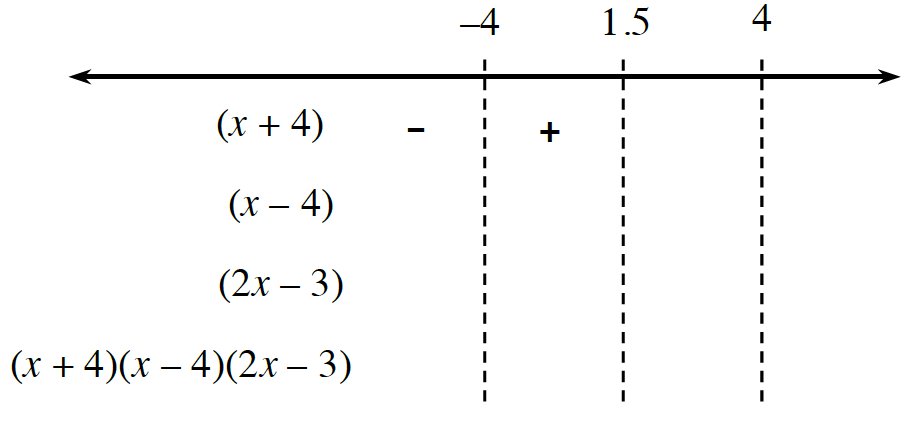### Home > PC3 > Chapter 5 > Lesson 5.2.2 > Problem5-76

5-76.

Solve each of the following inequalities.

1. $2x^3−3x^2>32x−48$

$2x^3-3x^2-32x+48>0$

$x^2(2x-3)-16(2x-3)>0$

$(x^2-16)(2x-3)>0$

$(x+4)(x-4)(2x-3)>0$

boundary points: $x=−4,4,1.5$

Complete a sign chart like the one below to determine the interval(s) where the expression is positive and negative.1. $\frac{x-10}{2x+7}\le-4$

$x\ne-\frac{7}{2}$

$\frac{x-10}{2x+7}+4\le0$

$\frac{x-10+4(2x+7)}{2x+7}\le0$

Simplify the numerator.
Use the factors in both the numerator and denominator to determine the boundary points.
Create a sign chart like the one shown in the steps for part (a).